# AP Board 9th Class Physical Science Important Questions Chapter 2 Laws of Motion

AP State Syllabus AP Board 9th Class Physical Science Important Questions Chapter 2 Laws of Motion

## AP State Syllabus 9th Class Physical Science Important Questions 2nd Lesson Laws of Motion

### 9th Class Physical Science 2nd Lesson Laws of Motion 1 Mark Important Questions and Answers

Question 1.
Calculate the momentum of a fast moving ball with a velocity 2.2 m/s of mass 6 kg.
mass (m) = 6 kg
velocity (v) = 2.2 m/s
momentum = mv = 6 × 2.2 = 13.2 kg.m.s-1
The momentum of a fast moving ball is 13.2 kg ms-1.

Question 2.
What is Newton’s first law of motion?
Every object will remains at rest or in a state of uniform motion, unless compelled to change its state by the action of a net force.Question 3.
From a fixed height, two eggs are dropped such that one falls on a concrete floor and the other on a cushioned pillow. The egg falling on the concrete floor breaks and the one falling on the pillow does not break. Explain why in terms of momentum.

• The change of momentum of an egg takes place in shorter time in case of concrete surface.
• The change of momentum of the egg takes place in longer time in case of cushioned pillow.
• Hence, egg does not break on cushioned pillow.

### 9th Class Physical Science 2nd Lesson Laws of Motion 2 Marks Important Questions and Answers

Question 1.
A vehicle of mass 300 kg travels at a velocity of 90 km/h. Find its momentum.
Mass of the vehicle (m) = 300 kg
Velocity of the vehicle (v) = 90 km/h = 90 × $$\frac{5}{18}$$ m/s = 25 m/s
Momentum = mv = 300 × 25 = 7500 kg.m.s-1Question 2.
“With our day to day experience, we must exert some force on an object to keep it moving”.
i) By which law, you can support the above lines?
ii) Which act on the body ? Either force or net force?
i) Newtons first law of motion.
ii) Net force. The position of object is changed due to net force only.

### 9th Class Physical Science 2nd Lesson Laws of Motion 4 Marks Important Questions and Answers

Question 1.
Fill the following table.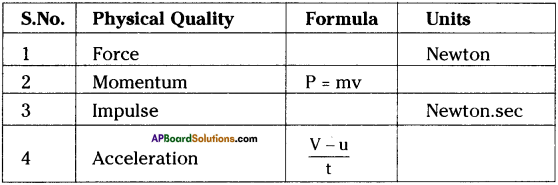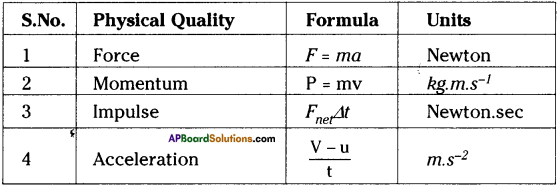Question 2.
What is impulse? Derive the formula for impulse.
Impulse :
The change of momentum of an object is called impulse.
Formulae for impulse ⇒ ∆p = Fnet∆t.

Derivation :
We can express the second law of motion as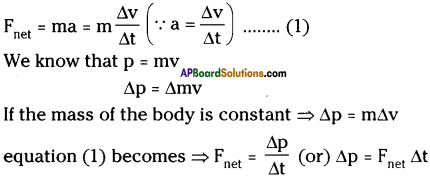From the above equation we know that the product of net force and interaction time is called impulse of net force (∆p).

Question 3.
State and prove the law of conservation of linear momentum.
Law of conservation of momentum : Law of conservation of momentum states, in the absence of a net external force on the system, the momentum of the system remains unchanged.
Explanation :

• Let two marbles with masses nij and m2 travel with different velocities ut and u2 in the same direction along a straight line.
• If u1 > u2, they collide each other and the collision lasts for time’t’.
• During collision, each marble exerts force on other marble. [F12 and F21]
• Let v1 and v2 be the velocities of the marbles after collision.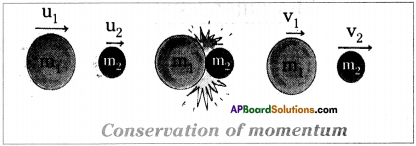Now look at the table.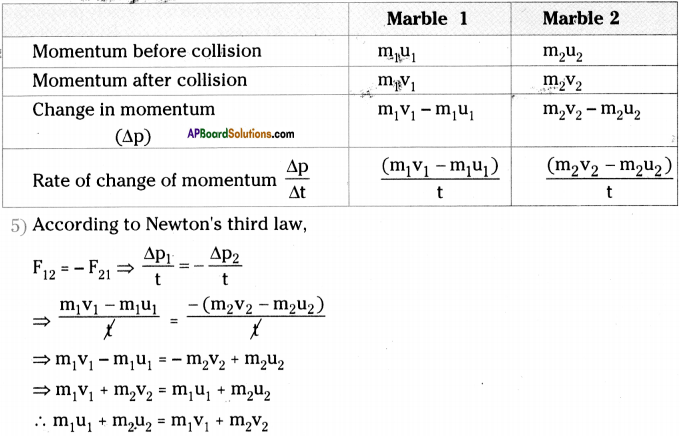• The total momentum before collision is equal to total momentum after collision.
• Hence, the total momentum remains unchanged before and after collision.

Question 4.
a) Name the ‘machine’ use to prove Newton’s laws of motion. Draw a neat diagram of it.
(OR)
The masses ‘m ‘ and ‘m2’ are attached to a string and roll over a pully, which is attached to a rigid support. Identify the machine and draw the neat diagram of it.
a) Atwood machine.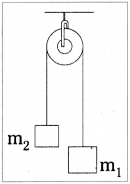b) Draw FBD of both, masses on it.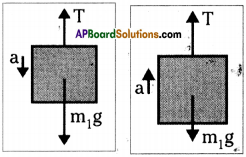9th Class Physical Science 2nd Lesson Laws of Motion 1 Mark Important Questions and Answers

Question 1.
Newton’s first law of motion is also called
Law of inertia.Question 2.
State Newton’s second law of motion.
The rate of change of momentum of a body directly proportional to the net force acting on it and it takes place in the direction of net force.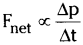Question 3.
What is inertia?
The natural tendency of objects to resist a change in their state of rest or of uniform motion is called inertia.

Question 4.
What is momentum?
Linear momentum of a body is the product of its mass and velocity.
Momentum (p) = mass (m) × velocity (v)

Question 5.
State Newton’s third law of motion.
When an object exerts a force on the other object, the second object exerts a force on the first one with equal magnitude and in opposite direction.

Question 6.
Write the law of conservation of momentum.
The law of conservation of momentum states that in the absence of net external force on the system, the momentum of the system remains unchanged.
m1u1 + m2u2 = m1v1 + m2v2

Question 7.
What is an impulse?
The product of net force and interaction time of a system is called impulse of net force.
Impulse ∆p = Fnet. ∆t.Question 8.
What is the Aristotle’s conclusion about the natural state of an object?
Aristotle concluded that the natural state of an earthly object is to be at rest. Hence the object at rest requires no explanation as any moving object naturally comes to rest.

Question 9.
What is the Galileo’s conclusion on the motion of an object?
Galileo gave birth to modern science by stating that an object in motion will remain in same motion as long as no external force is applied on it.

Question 10.
What is the effect of mass on inertia?
As mass increases, inertia increases.
Mass is a property of an object that specifies how much inertia the object has.

Question 11.
Who proposed laws of motion?
Sir Isaac Newton proposed the laws of motion.Question 12.
Give two daily life examples where you experience inertia.

• When the bus which is at rest begins to move suddenly, the person standing in the bus falls backward.
• When you are travelling in a bus the sudden stop of the bus makes you fall forward.

Question 13.
If action is always equal to the reaction, explain how a horse can pull a cart.
Horse and cart constitutes a single system. So they cannot form action-reaction pair. Therefore, horse can pull the cart.

9th Class Physical Science 2nd Lesson Laws of Motion 2 Marks Important Questions and Answers

Question 1.
What is momentum? What are its units in S.I. system?
Momentum (p) of a body is defined as the product of its mass (m) and velocity (v).
Momentum (p) = mass (m) × velocity (v)
p = mv
S.I. units of momentum are kg. m /sec (or) N – sec.

Question 2.
A mass of 0.5 kg has been suspended to a roof as shown in the figure. What is the force exerted by the rope on the object?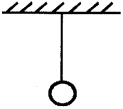The forces acting on the object of mass 0.5 kg are
i) gravitational force (downward) = 0.5 x 9.8 = 4.9 N
ii) Tension in the string T (upward).
The object is at rest. Hence the two forces are equal.
∴ The force exerted by the string on the object is 4.9 N (upwards).

Question 3.
Write few incidents if there were no friction.
(OR)
What happens when there were no friction in this world?
If there were no friction,

1. we could not be able to walk on ground.
2. we would not be able to stop a fast moving car.
3. any object could not be able to stay on the ground.Question 4.
What are the factors influencing acceleration? Explain.
Factors influencing acceleration are force and mass.
Force :
As the net force increases, the acceleration increases when mass remains constant.

Mass :
When force is constant, as the mass increases, the acceleration decreases.

Question 5.
Why does a fielder catch a fast moving cricket ball by pulling back his arms while catching it?
(OR)
Why does a cricketer move his hands backwards while catching a fast moving cricket ball?

• When he pulls back his arms he experiences a smaller force for a longer time.
• The ball stops only when your arms stop.
• This is to avoid a large impulse.
• If he doesn’t pull his arms back, the ball will hurt him.
• This is due to a larger force for a smaller time.

Question 6.
What is the state of an object when no net force is acting on an object?
If the net force acting on an object is zero, the object which is at rest remains at rest or if the object is already moving with a certain velocity it continue to move with same velocity.

Question 7.
Which of the following has more inertia?
a) A rubber ball and a stone of the same size.
b) A bicycle and a train.
c) A five rupees coin and a one-rupee coin.
As the mass of the object increases the inertia of the object increases.
a) Stone has more mass than rubber ball. So stone has more inertia.
b) Train has more inertia.
c) 5 rupee coin has more inertia.

Question 8.
Explain why some of the leaves may get detached from a tree, if we vigorously shake its branch?
The leaves are at the state of rest. When the tree is vigorously shaken the state of rest is disturbed. So the leaves are detached from the tree due to inertia of rest.Question 9.
Which would require a greater force – accelerating a 2 kg mass at 5 ms-2 or a 4 kg mass at 2 ms-2?
Force F = ma
Here we have m1 = 2kg, a1 = 5 ms-2 and m2 = 4 kg, a2 = 2ms-2.
Thus F1 = m1a1
Thus accelerating a 2 kg mass at 5 ms-2 would require a greater force.

Question 10.
A motor car is moving with a velocity of 108 km/h and it takes 4s to stop after the brakes are applied. Calculate the force exerted by the brakes on the motor car if its mass along with the passengers is 1000 Kg.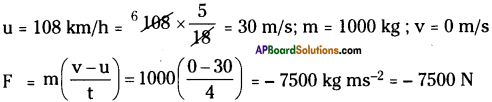Question 11.
Explain why is it difficult for a fireman to hold a hose, which ejects large amounts of water at a high velocity.
It is difficult for a fireman to hold a hose which ejects large amounts of water at a high velocity because due to high velocity of water the hose moves backward directiondue to Newton’s third law.

Question 12.
From a rifle of mass 4 kg, a bullet of mass 50 g is fired with an initial velocity of 35 ms-1. Calculate the initial recoil velocity of the rifle.
Mass of rifle m1 = 4 kg
Mass of bullet m2 = 50 g = $$\frac{50}{1000}$$ = 0.05 kg
Initial velocity of rifle u1 = 0
Initial velocity of bullet u2 = 0
Final velocity of rifle (recoil velocity) v1 = v
Final velocity of bullet v2 = 35 ms-1
According to law of conservation of momentum
m1u1 + m2u2 = m1v1 + m2v2.
4(0) + 0.05 (0) = 4v + 0.05 × 35
4v = – 1.75
v = – 0.44 m/s

Question 13.
An automobile vehicle has a mass of 1500 kg. What must the force between the vehicle and road if the vehicle is to be stopped with a negative acceleration of 1.7 ms-2?
m = 1500 kg ;
a = – 1.7 ms-2
F = ma = 1500 ×- 1.7 = -2550 NQuestion 14.
What happens to a person standing in a bus when the bus which is at rest begins to move suddenly?
(OR)
Explain static inertia with an example.

• The property of an object at rest will try to remain at rest is called “static inertia”.
• For example, when the bus which is at rest begins to move suddenly, the person standing in the bus falls backward because of static inertia of the body.
• Bus pulls the legs forward while body is in the rest position (static inertia).

Question 15.
Observe the given figure.
a) What happens to the coin, when we pull the paper with a jerk of force?
b) Why?1. The coin falls inside the glass.
2. This is due to static inertia of the coin.
3. This means coin in the state of ‘rest’ before and after pulling the paper. It falls down due to gravity only.

9th Class Physical Science 2nd Lesson Laws of Motion 4 Marks Important Questions and Answers

Question 1.
Give some examples for Newton’s third law of motion from your day-to-day observations.
1) Flying of birds :
a) When birds fly, they push the air downward with their wings.
b) The air pushes back the bird in opposite upward direction.
c) These two forces are equal in magnitude and opposite in direction. Hence the bird can fly.

2) Swimming of fish :
a) When a fish swims in water, it pushes back the water.
b) The water pushes the fish with equal but in opposite direction.
c) Hence the fish moves forward.

3) Launching of a rocket:
a) A rocket accelerates by expelling gas at high velocity.
b) The reaction force of the gas on the rocket accelerates the rocket in a direction opposite to the expelled gases.

Question 2.
Derive F = ma.
1) Newton’s second law of motion states that the rate of change of momentum of an object is proportional to the net force applied on the object in the direction of net force.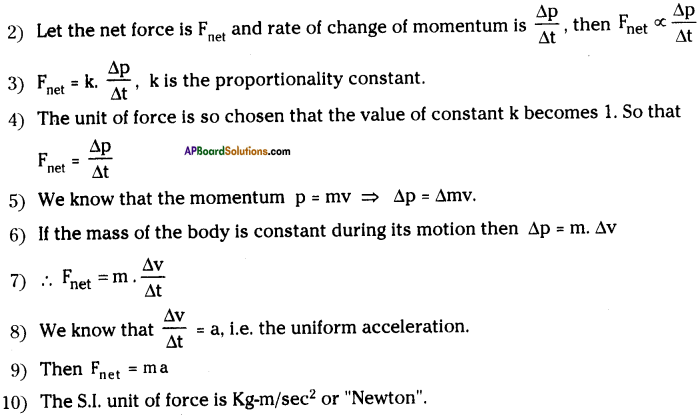Question 3.
How did Galileo differ the statement of Aristotle ‘The natural state of an earthy object is to be at rest?
(OR)
Write the great experiments of Galileo on inclined planes.
(OR)
How do you appreciate Galileo, the father of modern science?

• Galileo came up with two ingenious thought experiments.
• He did his experiments on inclined planes with smooth surfaces.
• He observed that the smoother the surface the farther the ball travelled.
• He extended this ajgument and concluded that if the surface was perfectly smooth, the ball will travel indefinitely until encountered by another object.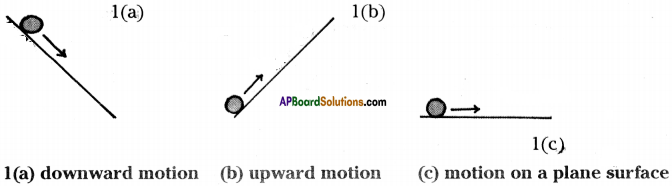• As shown in the figure 1 (a) he observed that when a marble rolls down a slope it picks up speed due to the force of gravity of the earth.
• As shown in the figure 1(b) when the object rolls up an inclined plane, its speed decreases.
• Now let us assume that a marble is moving on a level surface as shown in the figure 1(C), it has no reason to speed up or slow down.
• So, it will continue to move with constant velocity.
• By this experiment, Galileo came to a conclusion which was in contrast to Aristotle’s belief that the state of an earthy object is to be at rest.

Question 4.
Explain Newton’s third Law of motion with an activity.
(OR)
Take two spring balances of equal calibrations. Connect the two spring balances and pull them in opposite direction. Does they show different readings? Why?
Newton’s third law of motion : When an object exerts a force on an other object, the second object also exerts a force on the first one which is equal in magnitude but opposite in direction.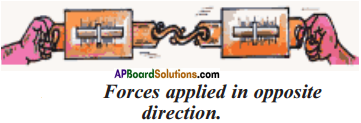Activity:

1. Let us take two spring balances of equal calibrations.
2. Connect the two springs balances as shown in the figure.
3. Pull the balances in opposite direction.
4. Observation : Spring balances shows same readings.
5. Here, one spring balance shows reading of ‘action’ force, and other spring balance shows reading of ‘reaction’ force.
6. Forces are equal, direction are opposite.
7. This shows Newton’s third law of motion.

Question 5.
Take two marbles of m1, m2 mass respectively. Make a collision in one second with marbles using u1, u2 initial velocities respectively. Take the final velocities as v1, v2 You observed the following in the experiment.
m1 = 10 kg u1 = 5 m/s v1 = 15 m/s
m2 = 100 kg u2 = 2 m/s -v2 = 1 m/s
t = 1 sec
Fill the table with suitable answers and write the conclusion from it.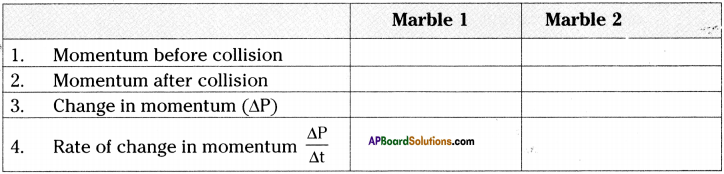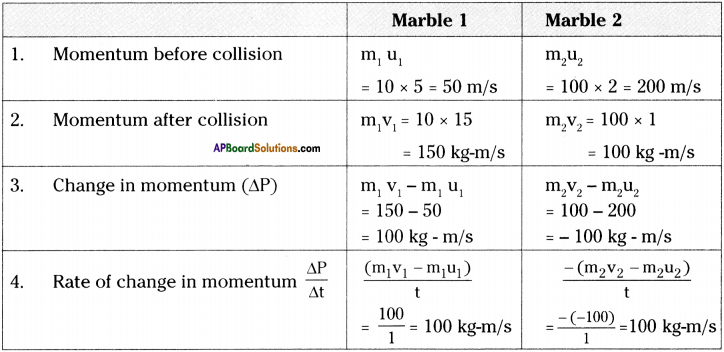Conclusion :

1. The table momentum is unchanged before and after collision.
2. We can say that the momentum is conserved.

Question 6.
How do you appreciate ‘Laws of motion’.
(OR)
How do you appreciate Newton, the great scientist.

1. Built upon ideas primarily developed by Aristotle and Galileo, Sir Isaac Newton proposed his three fundamental Laws.
2. These Laws explain the connection between force and a change in motion.
3. These Laws are popularly known as Newton’s Law of motion.
4. Newton’s first law of motion : A body continuous its state of rest or of uniform motion unless a net force acts on it.
5. This law is very useful to study about inertia, mass, weight of an object.
6. This law is used to prepare cranes, machines, vehicles, etc.
7. Newton’s second Law of motion : The rate of change of memontum of a body is directly proportional to the net force acting on it and it takes place in the direction of net force.
8. This law is useful to find the attraction between two objects.
9. It is very useful to study the behaviour of substances like velocity, acceleration, force, momentum, etc.
10. Newton third law of motion : If an object exerts a force on an other object, the second object also exerts a force on the first one which is equal magnitude but opposite in direction.
11. This law is used to prepare rockets.
12. In this way Newtons Law’s are very useful in the fields of automobiles space centres and various industries.
13. In this way Newton laws changed the life of human beings.
14. So, I am appreciating the laws of motion and Newton for his great service to us.

Question 7.
Write suitable law and concept to the given situation or vice versa in the given table.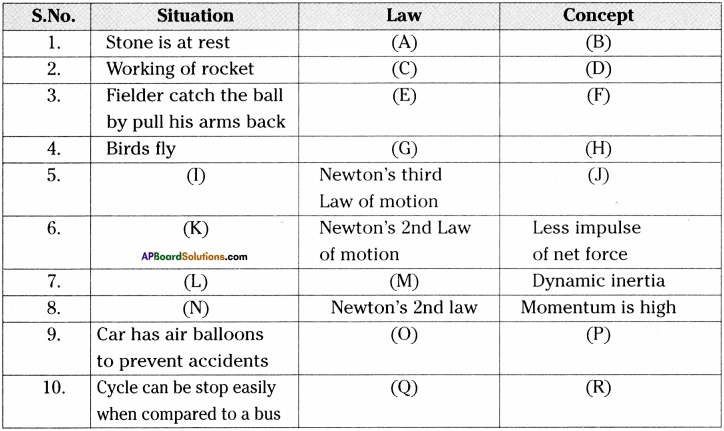A = Newton’s first law of motion
B = Static inertia
C = Newton’s third law of motion
D = Force of action = force of reaction
E = Newton’s second law of motion
F = Less impulse of net force
G = Newton’s third law of motion
H = Force of action = force of reaction
I = Fish swims in water
J = Force of action = force of reaction
K = Dropping egg on a cushion
L = Object thrown in universe (No external forces acts)
M = Newton’s first Law of motion
N = Bullet hurts
O = Newton’s second law of motion
P = Less impulse of net force
Q = Newton’s Second law of motion
R = Less momentum